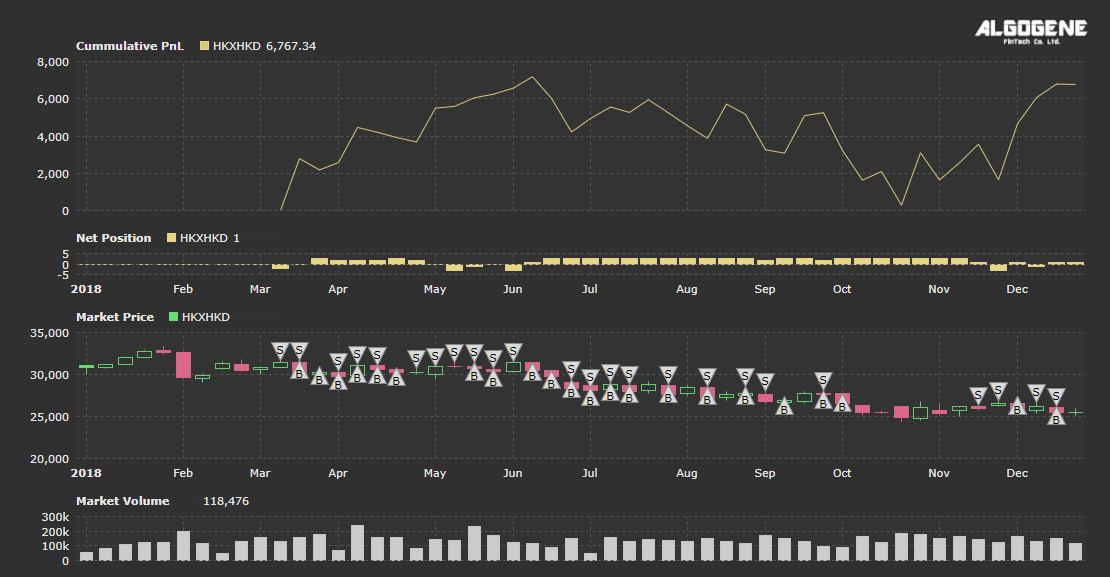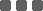## Stock Forecast - Autoregressive Model

This article discuss the implementation of Auto-Regressive (AR) Model as a forecasting trading strategy, then backtest the preformance in ALGOGENE Research Lab.

### Library to use:

The discussion below adopts a widely used Statistics Python package 'statsmodels'.

### Modeling Instruction:

In addition to estimating the parameters of a model, the library can also do forecasting, both in-sample and out-of-sample using 'statsmodels'. The in-sample is a forecast of the next data point using the data up to that point, and the out-of-sample forecasts any number of data points in the future. These forecasts can be made using either the predict() method if you want the forecasts in the form of a series of data, or using the plot_predict() method if you want a plot of the forecasted data. You supply the starting point for forecasting and the ending point, which can be any number of data points after the data set ends.

• Import the class 'ARMA' in the module 'statsmodels.tsa.arima_model'
• Create an instance of the ARMA class called 'mod' using the backtest data series data_1 and the order (p,q) of the model (in this case, for an AR(1) order=(1,0)
• Fit the model mod using the method '.fit()' and save it in a results object called res
• Start the forecast 5 data points before the end of the 30 point series at 25, and end the forecast 5 data points after the end of the series at point 35

Suppose we apply an AR(1) for daily Hang Seng Index CFD closing price, over the year of 2018.

• Based on a sliding window approach to collect the last 30 closing price
• Fit an AR(1) model, and forecast the next 5 closing price
• Submit a buy order if the 5th forecast price is above current level; else submit a sell order
• Set the holding period to 5 days for each position opening
• Repeat the process until the backtest period end

### Full Source Code:

 ``` 1 2 3 4 5 6 7 8 9 10 11 12 13 14 15 16 17 18 19 20 21 22 23 24 25 26 27 28 29 30 31 32 33 34 35 36 37 38 39 40 41 42 43 44 45 46 47 48 49 50 51 52 53 54 55 56 57 58 59 60 61 62 63 64 65 66 67 68 69 70``` ```from AlgoAPI import AlgoAPIUtil, AlgoAPI_Backtest from datetime import datetime, timedelta # Import the ARMA module from statsmodels from statsmodels.tsa.arima.model import ARIMA class AlgoEvent: def __init__(self): self.lasttradetime = datetime(2000,1,1) self.numOfData = 30 self.numOfForecast = 5 self.data_1 = [] def start(self, mEvt): self.myinstrument = mEvt['subscribeList'] self.evt = AlgoAPI_Backtest.AlgoEvtHandler(self, mEvt) self.evt.start() def on_marketdatafeed(self, md, ab): # check if it is a new day if md.timestamp > self.lasttradetime + timedelta(hours=24): # append last closing price self.data_1.append(md.lastPrice) # check if data_1 contain sufficient data point if len(self.data_1)>self.numOfData: # keep only the latest observation self.data_1 = self.data_1[-self.numOfData:] # base on AR(1) to make prediction val = self.nextMove() # print out result to console self.evt.consoleLog(md.timestamp, val) # send a buy order if val>0: self.sendOrder(1, 'open') # send a sell order elif val<0: self.sendOrder(-1, 'open') # update timer self.lasttradetime = md.timestamp def nextMove(self): # fit AR(1) model mod = ARIMA(self.data_1, order=(1,0,0)) res = mod.fit() # forecast the next arrPred = res.predict(start=self.numOfData, end=self.numOfData+self.numOfForecast) # calculate the distance between the 5th forecasted Price and current price currentPrice = self.data_1[-1] forecastPrice = arrPred[-1] return forecastPrice-currentPrice def sendOrder(self, buysell, openclose): orderObj = AlgoAPIUtil.OrderObject() orderObj.instrument = self.myinstrument orderObj.holdtime = 5*24*60*60 #unit in second, set maximun holding time to 5 days orderObj.volume = 0.01 orderObj.openclose = openclose orderObj.buysell = buysell orderObj.ordertype = 0 #0=market_order, 1=limit_order self.evt.sendOrder(orderObj) def on_orderfeed(self, of): pass def on_dailyPLfeed(self, pl): pass def on_openPositionfeed(self, op, oo, uo): pass ```

### Result:### Categories

Hot Topic

Guideline for Performance Report Generator

161 0 1 2023-09-18

What is the best method to extrapolate financial time series?

99 2 0 2023-09-11

A Python Example to Publish Trading Signal using REST API

235 1 3 2023-09-01

397 1 7 2023-08-25

What percentage of investor really make money?

205 3 1 2023-08-17

286 0 7 2023-08-07

Order closed with unexpected price

179 2 0 2023-07-26

Understand the difference between Pip, Point, Tick

196 0 1 2023-07-16